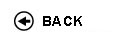Politecnico di Torino
Academic Year 2017/18
01NLBJM, 01NLBLI
Experimental statistics and mechanical measurement
1st degree and Bachelor-level of the Bologna process in Mechanical Engineering - Torino
1st degree and Bachelor-level of the Bologna process in Automotive Engineering - Torino
 Teacher Status SSD Les Ex Lab Tut Years teaching Galetto MaurizioO2 ING-IND/16 42 9 9 0 5
 SSD CFU Activities Area context ING-IND/12 6 B - Caratterizzanti Ingegneria meccanica
 Subject fundamentals The course aims to provide: - The basic concepts of probability and statistics used to manage and analyze the results of experiments; - The ability to understand general information about the features in the field of metrology tools and measuring system. - Knowledge concerning the elaboration and presentation of measurement and test results - The functional description of the main measuring instruments used in the mechanical (dimension, mass, force, strain, hardness, pressure and flow measurements). The topics will be exposed to the principles of the major national and international standards. Expected learning outcomes Knowledge of statistical distributions used in the field of measurement and quality Knowledge of the techniques of analysis of experimental data: regression hypothesis testing, ANOVA, exclusion principle Knowledge of the main metrological characteristics of measuring instruments: resolution, stability. repeatability, reproducibility, accuracy, the traceability chain. Knowledge of methods for evaluation of measurement uncertainty in accordance with national and international. Knowledge of major national and international standards in the field of metrology. Knowledge of the main operating principle of measuring instruments used in mechanics. Ability to set measurement activities by choosing the appropriate measuring instruments. Ability to perform the calibration of measuring instruments. Ability to detect systematic errors in the measurements. Ability to present the results of measurements and tests as specified in international standards. Ability to analysis of experimental results using statistical techniques (regression, ANOVA) Prerequisites / Assumed knowledge Concepts of Mathematics (calculation of derivatives), Concepts of Physics (knowledge of the main physical properties, units, etc.), and good practice in use of spreadsheets Contents INFORMATION CONTAINED IN MEASUREMENT RESULTS Guidelines on conformance with tolerances, management of measurement and test activities according to quality rules QUALITY MANAGEMENT OF MEASUREMENT AND TESTING Main principles of ISO 9001:2008 and ISO/IEC 17025:2005, dissemination of the International System of Units (SI) CHARACTERISTICS OF MEASURING INSTRUMENTS Resolution, stability, repeatability, reproducibility, bias, uncertainty, random errors, systematic errors and measurement accidents NOTES ON PROBABILITY The classic and frequentist concepts of probability, combinatorial analysis, compound and conditional probabilities NOTES ON STATISTICS Statistical distributions and relevant parameters, discrete distributions (binomial, hypergeometric and Poisson distributions), continuous distributions (normal, Student, Pearson and Fisher distributions) STATISTICAL MANAGEMENT OF EXPERIMENTAL DATA Description of experimental data, exclusion principles, frequency distributions, test of the χ� and Normal Probability Plot (NPP) THE LINEAR REGRESSION Least squares method, evaluation of the model goodness IDENTIFICATION OF SYSTEMATIC FACTORS The use of hypothesis tests, introduction to the analysis of variance (ANOVA) UNCERTAINTY OF TYPE B Evaluation of some uncertainty causes, the equivalent variance (uniform, arcsine and triangular distributions) THE COMBINED UNCERTAINTY Composition of the uncertainty contributions, the table of the uncertainty budget THE EXPANDED UNCERTAINTY The evaluation of degrees of freedom, practical applications and PUMA method ELEMENTS OF MECHANICAL MEASUREMENTS The length measurements (temperature and force effects, interactions between measurement surfaces and measurand), the mass measurements (effects of air density and gravity acceleration), the pressure measurements (basic concepts and devices based on weight force). Delivery modes The theoretical lessons on "Applications of Probability and Statistics", "Analysis of experimental data" and "Evaluation of measurement uncertainty" are followed by practical lessons in which the presented concepts are directly applied by the students to real problems. Texts, readings, handouts and other learning resources The reference textbook for the parts on "Applications of Probability and Statistics", "Analysis of experimental data" and "Evaluation of measurement uncertainty" is: G. Barbato, A. Germak, G. Genta, "Measurements for Decision Making", Societ� Editrice Esculapio, Bologna, 2013. For the part on "Elements of mechanical measurements", some slides will be distributed to the students. Assessment and grading criteria The exam consists of a written test subdivided into four parts: "Applications of Probability and Statistics", "Analysis of experimental data", "Evaluation of measurement uncertainty" and "Elements of mechanical measurements". For the first three parts, the students have to solve exercises similar to those carried out during the practical lessons. Instead, the part on "Elements of mechanical measurements" consists of a series of multiple choice questions (true/false): correct answers give points, while incorrect answers take away points. The time available for the exam is, indicatively, 2.5 hours. The exam, usually, takes place at LAIB and it is required to the students to use the PC assigned to them to carry out the examination. During the examination the students have available some files of the practical lessons carried out during the course, from which they can copy the calculation schemes. During the examination, it can be used the reference textbook of the course, but not notes or other material of any kind. The examination results will be published on "Portale della Didattica", in the section "Materiale", and after a day these will be officially registered and no longer modifiable. Programma definitivo per l'A.A.2016/17© Politecnico di Torino
Corso Duca degli Abruzzi, 24 - 10129 Torino, ITALY